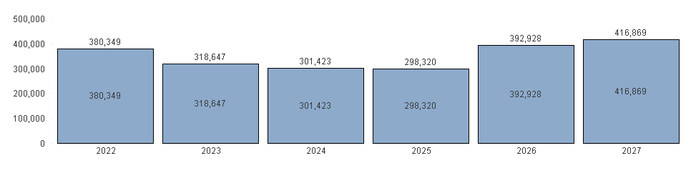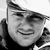# QlikView App Dev

Discussion Board for collaboration related to QlikView App Development.

Announcements
cancel
Showing results for
Did you mean:Contributor III

## Variables in Bar Charts

I have a the chart below that shows Demand by year.  I would like to have the ability to add/remove demand by year to see what the chart looks like after changes.  I have created 6 input boxes that create 6 unique variables, one for each year, and I would like to add a different amount into each input box/variable and have the chart update accordingly.The base expression to get the demand is : (Value is the demand dimension)

=sum(Distinct Value)

I can get it to add the same variable input in all years by updating to the following: (vIncrease is the variable created by the input box)

=sum(Distinct Value)+(vIncrease)

I would like each variable to impact only a specific year in the chart so I tried the expression below:

=sum(Distinct Value)+if(Year='2022',(vIncrease),if(Year='2023',(vIncrease123),if(Year='2024',(vIncrease124),if(Year='2025',(vIncrease125),if(Year='2026',(vIncrease126),if(Year='2027', (vIncrease127),0))))))

This does not work.  The results are the same as before I did any work with variables.  I have tried removing the single quotes from the year, I put the string inside the original sum equation, I have added each if statement as a separate added amount and not embedded.  Any thoughts on how to make this work, or if it is possible?

Labels (3)

• ### Variables

2 RepliesMVP

You could try to dollar-expand your variables like this \$(vIncrease).

=sum(Distinct Value) + if(Year='2022',\$(vIncrease),
if(Year='2023',\$(vIncrease123),
if(Year='2024',\$(vIncrease124),
if(Year='2025',\$(vIncrease125),
if(Year='2026',\$(vIncrease126),
if(Year='2027', \$(vIncrease127),0))))))

Does this help?Contributor III
Author

Thanks for the suggestion.  that did not work for me, still just like the original chart with no variable add